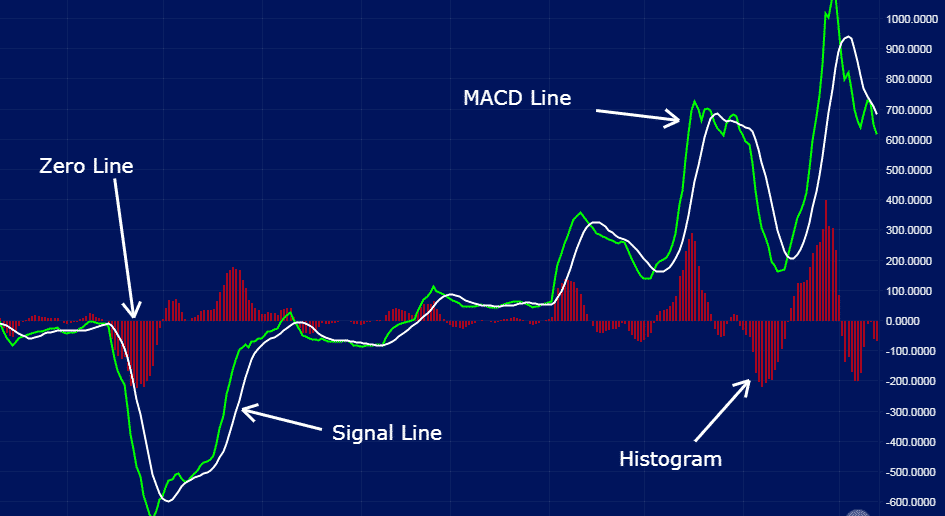## MACD Above Zero Line: What It Means In 2021

Today we are going to look at which it means to have the macd above zero line.

Can we use it as a reliable indicator for long or short trades?

Moving Average Convergence Divergence is a widely used indicator for analyzing market momentum.

While it also doubles as an oscillator, it is not commonly used to identify overbought and oversold conditions.

Instead, it is used to identify trends.

The indicator uses two moving averages tries to predict the formation of a new trend.

### MACD Indicator Description

The indicator appears in charts as two lines; the MACD line and the signal line.

There is also a zero line, above which and below which underlying trends emerge.

As the two moving average lines cross each other, they give rise to crossover patterns that traders look to profit from.Source: Blog.liquid.com

The two lines oscillate without boundaries.

The shorter line is usually a 12-period Exponential Moving Average that moves quicker.

The longer one is usually a 26 Exponential Moving Average that moves slower.

Additionally, the MACD indicator comes with a histogram, which indicates the number of bars used to calculate the moving average and the difference between the faster and slower moving averages.

### Understanding The MACD Indicator

The MACD line is usually the difference between the two exponential moving averages, 12 and 26.

It also represents the difference between the two lines.

In the MACD indicator, the MACD line is usually the faster-moving average.

On the other hand, the Signal Line is usually the slower moving average.

The Signal line plots the average of the previous MACD line.

In most cases, it is usually the 9-period exponential moving average.

The signal line is used to smooth the sensitivity of the MACD line.

On the other hand, the histogram plots the difference between the MACD line and the Signal line, representing the same in bars.

Depending on how the bars form, they can signal a crossover is about to happen.

Disclaimer: The information above is for educational purposes only and should not be treated as investment advice. The strategy presented would not be suitable for investors who are ...

more